﻿ Disc under Distributed LoadExamples | Product | Murata Software Co., Ltd.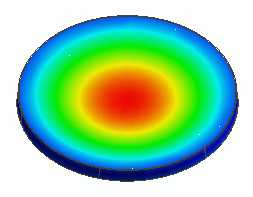General

• Distributed load is applied on a metal disc

• The deformation, the displacement and the mechanical stress are solved.

• Unless specified in the list below, the default conditions will be applied.

Analysis Space

 Item Settings Analysis Space 3D Model unit mm

Analysis Conditions

This is a static analysis.

 Item Settings Solvers Mechanical Stress Analysis [Galileo] Analysis Type Static analysis Options N/A

Model

The disc is a low-height cylindrical solid body. The material is copper.

Distributed load is applied on the top face. The bottom circumference is fixed.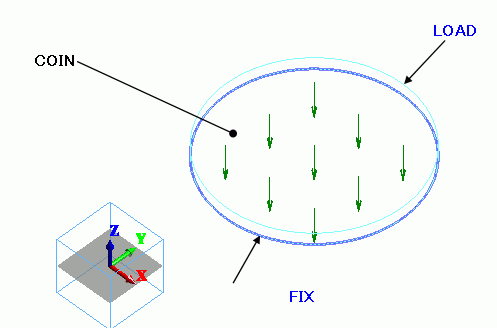Body Attributes and Materials

 Body Number/Type Body Attribute Name Material Name 0/Solid COIN 008_Cu *

* Available from the Material DB

Boundary Conditions

The total load applied on the top face is 980N (100kgf) which is distributed uniformly on the face.
Face area: (23.5/2)2×3.14=433.5 mm2

Total load: 10×9.8 = 98 N

Distributed face load: Total load / Face area = 2.26×105 N/ｍ2  (=Pa)

 Boundary Condition Name/Topology Tab Boundary Condition Type Settings FIX/Edge Mechanical Displacement Select the Z component. UZ=0 LOAD/Face Mechanical Distributed face load X=0, Y=0, Z=-2.26×10^5[Pa]

Results

The Z-direction displacement is shown below by contour. The contour is the Z displacement.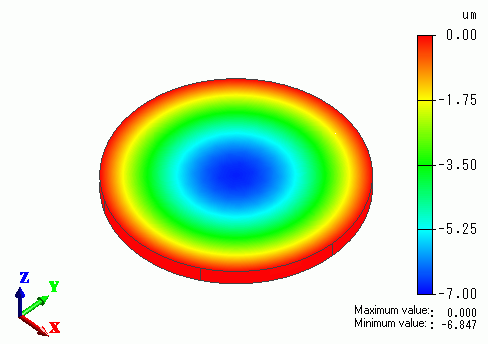The center area is sinking approximately 7um.

The vectors of the mechanical stress are shown below.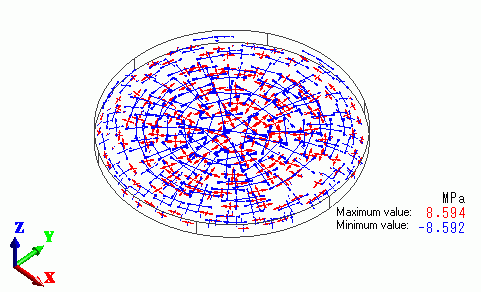The upper side is getting the tensile stress, whereas the lower side is getting the compression stress.

The external force and reactive force are listed in the table below.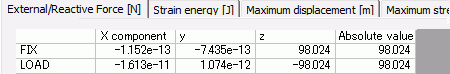The reactive force at FIX is 98[N] in +Z direction.

The external force at LOAD is 98[N] in -Z direction.

﻿